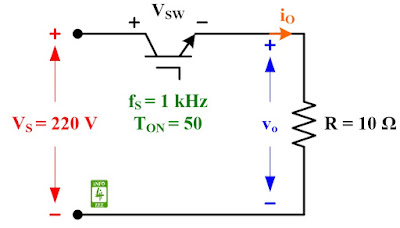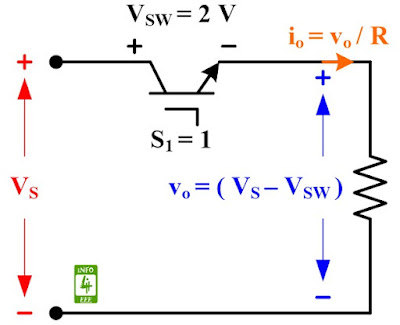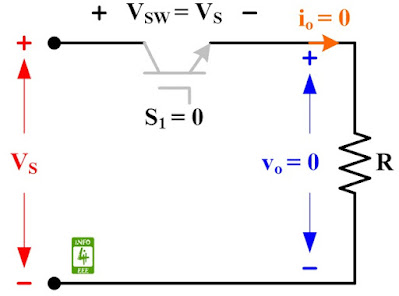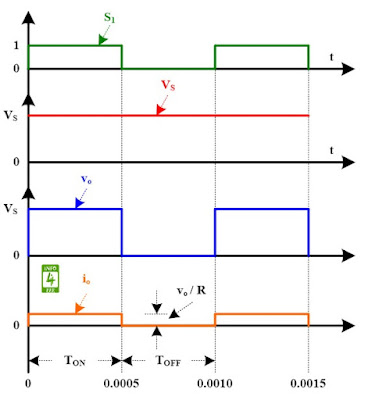Step down chopper is used to step down the dc voltage. The circuit diagram of step down chopper with resistive load is given in Fig. 1. The step-down chopper has input voltage of 220 V and resistance of 10 Ω. The switch (S1) is operated with signal of 1 kHz switching frequency and 50 % duty cycle.Fig. 1 Circuit diagram of step-down chopper with resistive load.
Step-down chopper operates in two mode.

Mode-1: When the switch is ON, the switch voltage, VSW is 2 V, output voltage, vo is (VS - VSW), and output current, io is (vo /R).Fig. 2 Equivalent circuit of step-down chopper with resistive load during mode-1.

Mode-2: When the switch is OFF, the output voltage and current are zero.Fig. 3 Equivalent circuit of step-down chopper with resistive load during mode-2.
Waveform showing switching signal (S1), input voltage (VS), output voltage (vo), and output current (io) for step down chopper with resistive load is given in Fig. 4.Fig. 4 Waveforms for step-down chopper with resistive load.
The average and RMS value of output voltage for step-down chopper can be obtained with following formulas.

Average Output Voltage, Va = (V- VSW) ✕ ( TON / (TON+TOFF) )
Va = (220 - 2) ✕ ( 0.0005 / (0.0005+0.0005) )
Va = (220 - 2) ✕ ( 0.5 ) = 109 V

RMS Output Voltage, VO(V- VSW) √ (TON/(TON+TOFF))
VO = (220 - 2) √ (0.5) = 154.15 V

#### Reference

M. H. Rashid, “Power Electronics: Circuits, Devices and Applications,” Prentice Hall India, Second Edition, 2006.